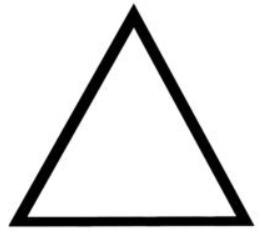Courses

# Test: Shapes (Geometry) - 1

## 10 Questions MCQ Test Mathematics for Class 3: NCERT | Test: Shapes (Geometry) - 1

Description
This mock test of Test: Shapes (Geometry) - 1 for Class 3 helps you for every Class 3 entrance exam. This contains 10 Multiple Choice Questions for Class 3 Test: Shapes (Geometry) - 1 (mcq) to study with solutions a complete question bank. The solved questions answers in this Test: Shapes (Geometry) - 1 quiz give you a good mix of easy questions and tough questions. Class 3 students definitely take this Test: Shapes (Geometry) - 1 exercise for a better result in the exam. You can find other Test: Shapes (Geometry) - 1 extra questions, long questions & short questions for Class 3 on EduRev as well by searching above.
QUESTION: 1

### Which among the following is not an example of a plane?

Solution:

bottle has no boundary, where the surface stops.

QUESTION: 2

Solution:
QUESTION: 3

### Consider on above figure shown below: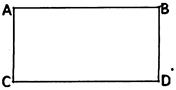Based on above figure, which one among the following is not correct?

Solution:
QUESTION: 4

Identify the figure in which incorrect name of the figure is shown?

Solution:

In option 3, the figure shown is cone not circle.

QUESTION: 5

Which one of the following statements is incorrect?

Solution:

Rectangle has 4 vertices not 5

QUESTION: 6

Directions: See each of the following figures and choose the correct name of the shape.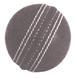Solution:
QUESTION: 7

Directions: See each of the following figures and choose the correct name of the shape.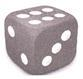Solution:
QUESTION: 8

Directions: See each of the following figures and choose the correct name of the shape.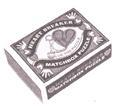Solution:

A match box is made from rectangles and the only 3-d shape that is made out of rectangles is cuboid.

QUESTION: 9

Directions: See each of the following figures and choose the correct name of the shape.
A cube has ________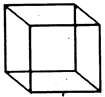Solution:
QUESTION: 10

Mark the correct statement.

Solution:

Triangle has three sides.